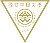# 最新消息

##演講通知-Prof. Tibor Krisztin 2023/09/13(三) 14:30-16:00

### 2023年09月12日

https://ntucc.webex.com/ntucc-en/j.php?MTID=m771f170721d43eb71ea278befa264331

Meeting number (access code): 2515 027 2890

Abstract：

Delay differential equations of the form

\$y'(t)=-ax(t)+bh(y(t-1))\$

with positive real parameters a, b and a nonlinear function \$h: [0, infty ) o [0, infty )\$ arise in modelsfor the dynamics of single species populations.

For monotone nonlinearities h the dynamics is relatively simple: There is a Morse decomposition of the global attractor, on each Morse component the dynamics is planar, and a fine structure of the global attractor can be described.

A nonmonotone, in particular a hump-shaped, or unimodal nonlinearity h can cause entirely different dynamics. An example  is the hump-shaped \$h(xi)=displaystyle frac{xi^k}{1+xi^n}\$  ,  where k, n are positive parameters.

The case k=1  was proposed in 1977 by  Mackey and  Glass as a model for the feedback control of blood cells. Since then this particular equation attracted the attention of many mathematicians interested in nonlinear dynamics and delay differential equations.

The case k=2 was introduced by Morozov, Banerjee and Petrovskii in 2016 as a minimal model to account for some of the most common features of population ecology whilst it

remains capable of simulating sufficiently complex dynamics including long-living transients and chaos. Despite the large number of results showing convergence, oscillations, bifurcations, and complicated solution behavior, the dynamics is not understood yet. Most of the results are numerical.

We prove that for each \$k geq 1\$ there are parameter values b>a>0 such that, for sufficiently large n, orbitally asymptotically stable periodic orbits exist. The periodic orbits can be complicated in the sense that the projections \$mathbb{R} i t o (x(t), x(t-1)) in mathbb{R}^2\$ can produce complicated looking figures.

In the case k>1 an additional equilibrium point \$xi^*\$ arises, comparing to the case k=1 , from  which there exist connecting orbits to zero and to the stable periodic orbit obtained in the first step. Heteroclinc connections are shown between periodic orbits.  Moreover, we rigorously prove the existence of homoclinic orbits to \$xi^*\$ .